# Unit conversion - 7th grade (12y) - math problems

#### Number of problems found: 462

• The Nürburgring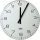The Nürburgring in Germany is a racetrack that hosts racing events all year. Two of the fastest laps ever driven on the track are 6 minutes 47.30 seconds and 6 minutes 52.01 seconds. How much faster is the 6 minutes 47.30 seconds time than the 6 minutes 5
• Temperatures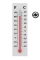Temperatures in degrees Fahrenheit and Celsius are related by the rule F = 1.8C + 32. What is the temperature in degrees Celsius if it is 50°F?
• Gas priceIf a cm3 of gas costs rm 1.50, how many cents would a liter of gas cost? 1 rm = 1 Malaysian Ringgit = 100 Malaysian Ringgit cents = equals 0.21 Euro in 2021/Q3
• I-beam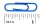If an I-beam is 12’- 6  1/2” long, and it needs to be cut into 4 equal sections, how long will each section be?
• Central Park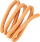Central Park is a rectangular park in New York City and the map scale is 1 cm: 320 m (It means 1 cm on the map represents 320 m in the real world. ). Find the north-south distance if the length on the map is 2.5 cm.
• ParallogramThe Parallelogram base is 24 cm and high 10 cm. How many are such tiles required to cover a floor of area 1080m2?
• Licorice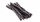A piece of licorice is 2/3 yard long.  If it is cut into 10 equal pieces, how long will each piece of licorice be?
• Cutting wireIf you cut a 3 ½ ft length wire into pieces that are 2 inches long, how many pieces of wire will you have?
• Ferdinand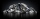Ferdinand went from place A to place B and from there to place C. A is 7.2 km from B and B is 11.7 km from C. Albert went from place A to place D and from there to place C. D is 9.1 km from A and C is 10.8 km from D. Who traveled more and by how much?
• Eights of butter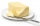How many eights of butter (1/8 of kg = 125 g) can be stored in a box with dimensions of 4 dm, 2 dm, 1.8 dm, if the eighth of butter has dimensions of 8 cm, 5 cm, 3 cm?
• A straight angle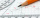A straight angle measures s, so you can subtract or add 85 from What are the measure of their angles?
• Each ofEach of Suzan's notebooks is 4/5  inches wide. If she has 25 inches of space remaining on her bookshelf, how many notebooks will fit?
• Two citiesOn a map, 1.5 cm represents 50 miles. If the distance between the two cities on the map is 6 cm, how many miles separate the cities?
• Grocery store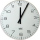You spend 4/5 of an hour at the mall. You spend 1/3 of that time in the food court. How many minutes are you in the food court?
• Little 2Little fields farm lies on a rectangular plot of land that measures 2 2/4 miles in length and 2 2/3 miles in width. What is it's area in square miles?
• A rope 2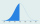A rope that is 6 meters long will be cut into 24 pieces that are all of the same length. What will be the length of each piece in centimeters? (100 cm = 1 m)
• A raindrop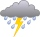A raindrop falls at a rate of 9.3 meters per second (m/s). Use the following facts to convert this speed to kilometers per hour (km/h). 1 km = 1000 m 1 min = 60 sec 1 hour = 60 min
• The physicalThe physical therapist asks Mr. Smith to walk 20 minutes in one hour to improve his ambulation. What fractional part of an hour is Mr. Smith to exercise?
• A map 4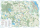A map represents every 4 miles with 1 inch. If a school and a bank are actually 12 miles apart, how far apart are they on the map, in inches?
• Tina has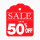Tina has 10 packages of yarn to knit a scarf. She uses 1/3 of package of yarn for each inch of length she adds to the scarf. If Tina uses all the yarn, how long will her scarf be?

Do you have an exciting math question or word problem that you can't solve? Ask a question or post a math problem, and we can try to solve it.

We will send a solution to your e-mail address. Solved examples are also published here. Please enter the e-mail correctly and check whether you don't have a full mailbox.

Unit conversion - math problems. Examples for 7th grade (seventh).